# Maharashtra Board Class 12 Chemistry Important Questions Chapter 9 Coordination Compounds

Balbharti Maharashtra State Board 12th Chemistry Important Questions Chapter 9 Coordination Compounds Important Questions and Answers.

## Maharashtra State Board 12th Chemistry Important Questions Chapter 9 Coordination Compounds

Question 1.
What are double salts?
Double salts are crystalline molecular or addition compounds containing more than one salt in simple molecular proportions soluble in water and in solution they ionise and exhibit all the properties of the constituent ions.

For example, K2SO4+ A12(SO4)324H2O
$$\mathrm{K}_{2} \mathrm{SO}_{4} \cdot \mathrm{Al}_{2}\left(\mathrm{SO}_{4}\right)_{3} \cdot 24 \mathrm{H}_{2} \mathrm{O}_{(\mathrm{aq})} \longrightarrow 2 \mathrm{~K}_{(\mathrm{aq})}^{+}+2 \mathrm{Al}_{(\mathrm{aq})}^{3+}+4 \mathrm{SO}_{4(\mathrm{aq})}^{2-}+24 \mathrm{H}_{2} \mathrm{O}_{0}$$Question 2.
Define coordination compound.
Coordination compound : It consists of a central metal ion or atom surrounded by atoms, molecules or anions called ligands by coordinate bonds, e.g. cisplatin Pt(NH3)2Cl2, [Cu(NH3)4]SO4.

Question 3.
Define Lewis bases and Lewis acids with respect to a coordination compound.

• Lewis bases : In a coordination compound the ligands being electron pair donors they are Lewis bases.
• Lewis acids : The central metal atom or ion being electron acceptor behaves as a Lewis acid.
• For example, in the coordination compound, [Cu(NH3)4]2+, NH3 is a Lewis base and Cu2+ is a Lewis acid.

Question 4.
Define coordination sphere. Give example.
Coordination sphere : A coordination entity consisting of a central metal atom or ion and the coordinating groups like neutral molecules or anions (ligands) written inside a square bracket is together called coordination sphere. This is a discrete structural unit. The ionisable groups (generally ions) called counter ions are written outside the bracket.

For example, in the coordination compound K4[Fe(CN)6], the coordination sphere is [Fe(CN)6]4- while K+ represents counter ion.

Question 5.
Define and explain charge number of a complexion.
Charge number of a complexion : The net charge carried by a complexion or a coordination entity is called its charge number.

Explanation :
(i) Charge number is equal to the algebraic sum of the charges carried by central metal atom or ion and all the ligands attached to it.
(ii) E.g. consider anionic complex, [Fe(CN)6]4-.
Charge number of [Fe(CN)6]4- = Charge on Fe2+ ions + 6 x charge on CN = ( + 2) + 6( -1) = – 4 Hence charge number of [Fe(CN)6]4- is – 4.

Question 6.
Explain the oxidation state of a metal in a complex.

• The oxidation state of a metal atom or ion in the complex is the apparent charge carried by it in the complex.
• It depends upon the atomic number and electronic configuration of the metal atom or ion.
• The coordination number, the formula and geometry of a complex depend upon the oxidation state of the metal
atom or ion.

Question 7.
What is the charge on a monodentate ligand X in the complex, [NiX4]2-?
The charge number of the complex ion is – 2. Nickel being divalent, its oxidation state is + 2. If the charge on monodentate ligand X is y, then Charge number = charge on Ni2+ charge on 6X – 2 = + 2 + 4 xy
∴ y = – 1
Hence the charge on ligand X is – 1.

Question 8.
Calculate the oxidation state of a metal in the following complexes :
(a) [Fe(NH3)6](NO3)3
(b) Ni(CO)5.
(a) [Fe(NH3)6](NO3)3 ⇌ [Fe(NH3)6]3+ + 3NO3

NH3 is a neutral ligand, and the charge number of complex ion is + 3.
If the oxidation state of Fe is x then,
+ 3 = x + 6(0)
∴ x = + 3
∴ The oxidation state of Fe is +3.

(b) Ni(CO)5 is a neutral complex and CO is a neutral ligand. If the oxidation state of Ni is x, then zero = x + 5 x (zero)
∴ x = zero.
The oxidation state of Ni is zero.

Question 9.
Define and explain the term coordination number (C.N.) of a metal in the complex.
Coordination number or legancy (C.N.) : The number of (monodentate) ligands which are directly bonded by coordinate bonds to central metal atom or ion in a coordination compound is called coordination number (C.N.) of the metal atom or ion.

Explanation :

• The coordination number (C.N.) is a characteristic property of the metal and its electronic configuration.
• C.N. takes the values from 2 to 10, of which 4 and 6 are very common.
• The light transition metals show C.N. 4 and 6 while the heavier transition metals show C.N. 8.
• The geometry and shape of a complex compound depends upon C.N. of the metal.Question 10.
Mention primary valence, secondary valence and coordination number in the following complexes :
(a) [Cu(NH3)JCI2
(b) [Co(NH3)3CI3]
(C) K4[Fe(CN)6]
(d) [CoF6]3
(e) [Pt(NH3)2Cl2]
(f) [Pt(NH3)2(Py)3CI2]
(g) Cr(CO)6
(h) [Ni(CN)4]2-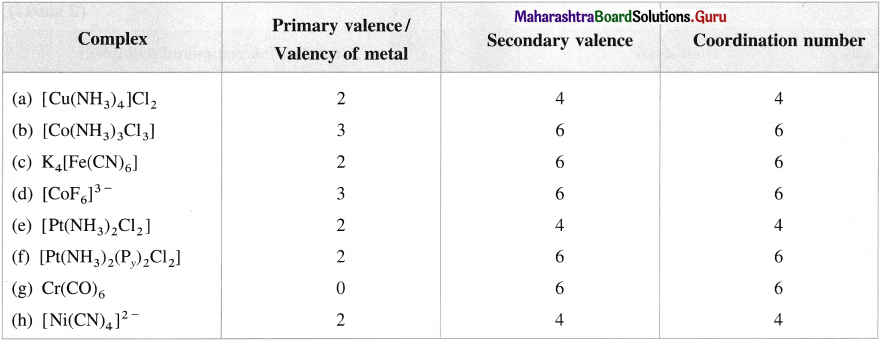Question 11.
Classify the following complexes as homoleptic and heteroleptic complex :
(a) [Cu(NH3)4]SO4;
(b) [Cu(en)2(H2O)CI]2+
(c) [Fe(H2O)5(NCS)]2+
(d) Tetraaminezinc(II) nitrate.

Question 12.
Summarise the rules of IUPAC nomenclature of coordination compounds.
Following rules are followed for naming coordination compounds recommended by IUPAC :

1. In case of a complexion or a neutral molecule, name the ligand first and then the metal.
2. The names of anionic ligands are obtained by changing the ending -ide to -o and -ate to -ato.
3. The name of a complex is one single word. There must not be any space between different ligand names as well as between ligand name and the name of the metal.
4. After the name of the metal, write its oxidation state in Roman number which appears in parentheses without any space between metal name and parentheses.
5. If complex has more than one ligand of the same type, the number is indicated with prefixes, di-, tri-, tetra-, penta-, hexa- and so on.
6. For the complex having more than one type of ligands, they are written in an alphabetical order. Suppose two ligands with prefixes are tetraaqua and dichloro. While naming in alphabetical order, tetraaqua is written first and then dichloro.
7. If the ligand itself contains numerical prefix in its name, then display number by prefixes bis for 2, tris for 3, tetrakis for 4 and so forth. Put the ligand name in parentheses. For example, (ethylenediamine)3 or (en)3 would appear as tris (ethylenediamine) or tris(ethane-l, 2-diamine).
8. The metal in cationic or neutral complex is specified by its usual name while in the anionic complex the name of metal ends with ‘ate’.

Question 13.
State effective atomic number (EAN).
OR
State and explain effective atomic number (EAN). How is it calculated?
Effective atomic number (EAN) : It is the total number of electrons present around the central metal atom or ion and calculated as the sum of electrons of metal atom or ion and the number of electrons donated by ligands.

It is calculated by the formula : EAN = Z – X + Y where.
Z = Atomic number of metal atom
X = Number of electrons lost by a metal atom forming a metal ion
Y = Total number of electrons donated by all ligands in the complex.

Generally the value of EAN is equal to the atomic number of the nearest inert element.

Explanation : Consider a complex ion [Co(NH3)6]3+
Oxidation state of cobalt is + 3 hence X = 3.
There are six ligands, hence Y = 2 x 6 = 12
Atomic number of cobalt. Z = 27
∴ EAN = Z – X + Y = 27 – 3 + 12 = 36.Question 14.
Find effective atomic number (EAN) in the following complexes :
(1) [Ni(CO)4]
(2) [Fe(CN)6]4-
(3) [Co(NH3)6]3+
(4) [Zn(NH3)J2+
(5) [Pt(NH3)6]4+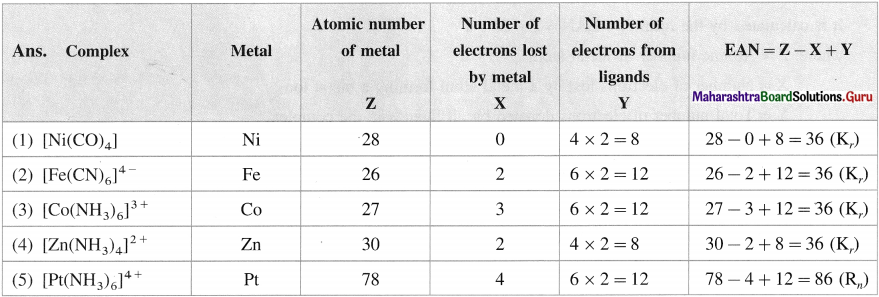Question 15.
What is effective atomic number (EAN) in the following complexes ?
(1) [Fe(CN)6]3-
(2) [CU(NH3)4]2+
(3) [Pt(NH3)4]2+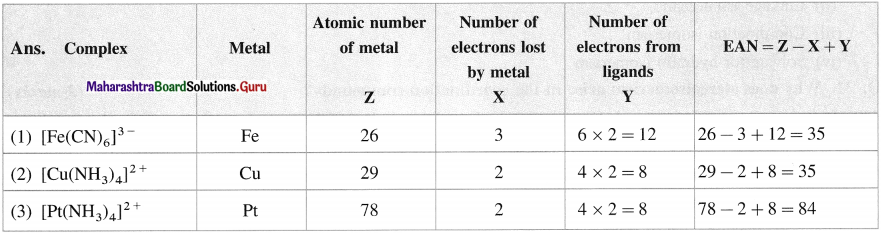Question 16.
Calculate EAN in the following complexes :
(1) [Cr(H2O)2(NH3)2(en)]CI3;
(2) [Ni(en)2]SO4;
(3) Na3[Cr(C2O4)3].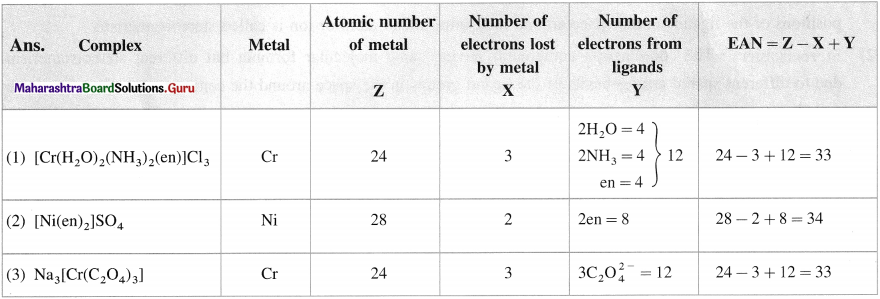Question 17.
Define in coordination compounds :
(1) Isomerism
(2) Isomers.

1. Isomerism : It is the phenomenon in coordination compounds having same molecular formula but different physical and chemical properties due to different arrangements of the ligands around the central metal atom or ion in the space.
2. Isomers : The isomers are the coordination compounds having same molecular formula but different physical and chemical properties due to the difference in arrangements of the ligands in the space.

Question 18.
Mention the types of isomerisms in coordination compounds.
There are two principal types of isomerisms in coordination compounds as follows :
(A) Stereoisomerism
(B) Structural isomerism (OR Constitutional isomerism)

(A) Stereoisomerism is further classified as :

• Geometrical isomerism
• Optical isomerism

(B) Structural isomerism is further classified as :

• Ionisation isomerism
• Coordination isomerism
• Solvate (or hydrate) isomerismQuestion 19.
Why does stereoisomerism arise in the coordination compounds?
In the coordination compounds (complexes) the ligands are linked to the central metal atom or ion by coordinate bonds which are directional in nature and hence give rise to the phenomenon of stereoisomerism.

In this isomerism, the different stereoisomers have different arrangements of ligands (atoms, molecules or ions) in space around the central metal atom or ion. Hence they have different physical and chemical properties and give rise to the phenomenon of stereoisomerism.

Question 20.
Define, in coordination compounds : (1) Stereoisomerism (2) Stereoisomers.
(1) Stereoisomerism The phenomenon of isomerism in the coordination compounds arising due to different spatial positions of the ligands in the space around the central metal atom or ion is called stereoisomerism.

(2) Stereoisomers : The coordination compounds having same molecular formula but different stereoisomerism due to different spatial arrangements of the ligand groups in the space around the central metal atom or ion are called stereoisomers.

Question 21.
Define :
(1) Geometrical isomerism and
(2) Geometrical isomers.
(1) Geometrical isomerism : The phenomenon of isomerism in the heteroleptic coordination compounds with the same molecular formula but different spatial arrangement of the ligands in the space around the central metal atom or ion is called geometrical isomerism.

(2) Geometrical isomers : The heteroleptic coordination compounds having same molecular formula but different geometrical isomerism due to different spatial arrangements of the ligands in the space around the central metal atom or ion are called geometrical isomers.

Question 22.
Define cis and trails isomers in the coordination compounds.
(1) Cis-isomer : A heteroleptic coordination compound in which two similar ligands are arranged adjacent to each other is called cis-isomer. For example,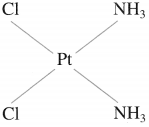Gis-Diamminedichloroplatinum(II)

(2) Trans-isomer : A heteroleptic coordination compound in which two similar ligands are arranged diagonally opposite to each other is called trans-isomer. For example,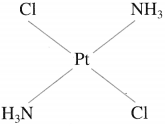Trans-Diamminedichloroplatinum(II)

Question 23.
Write structures for geometrical isomers of Diamminebromochloroplatinum(II).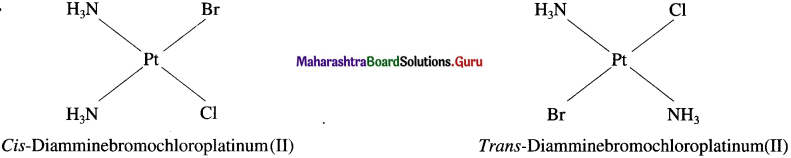Question 24.
Explain the geometrical isomerism of the octahedral complex of the type [MA4B2] with a suitable example.

• Consider an octahedral complex of a metal M with coordination number six and monodentate ligands a and b having formula [MA4B2],
• CA-isomer is obtained when both the B ligands occupy adjacent (1,2) positions.
• Trans-isomer is obtained when the ligands B occupy the opposite (1,6) positions.
• For example, consider a complex [CO(NH3)4CI2]+. The structures of cis and trans isomers are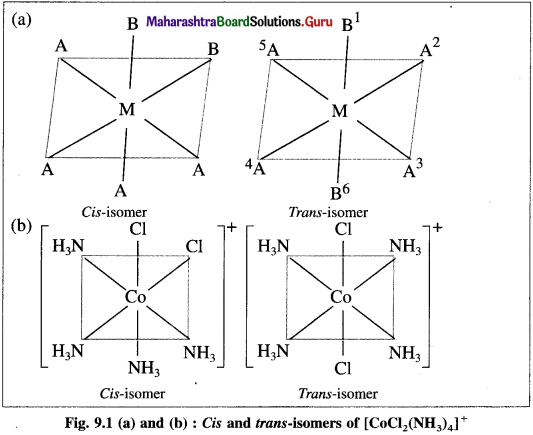Question 25.
Explain the geometrical isomerism of the octahedral complex of the type [M(AA)2B2] with a suitable example.

• Consider an octahedral complex of metal M with coordination number six and a bidentate ligand AA and monodentate ligand B having molecular formula [M(AA)2B2] .
• Bidentate ligand AA has two identical coordinating atoms.
• Cis- isomer is obtained when two bidentate AA ligands as well as two ‘B’ ligands are at adjacent positions.
• Trans-isomer is obtained when two AA ligands and two B ligands are at opposite positions.
• For example, consider a complex [Co(en)2CI2]+.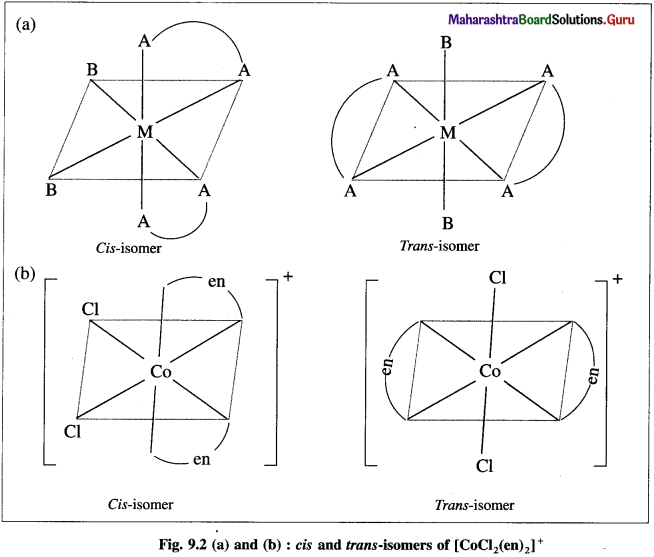Question 26.
Explain the geometrical isomerism of the octahedral complex of the type [MA4BC] with suitable example.

• Consider an octahedral complex of metal M with coordination number six and monodentate ligands A, B andC.
• Cis-isomer is obtained when both the ligands B and C occupy adjacent (1,2) positions.
• Trans-isomer is obtained when the ligands B and C occupy opposite positions.
• For example, consider a complex [Pt(NH3)4BrCI] of the type [MA4BC],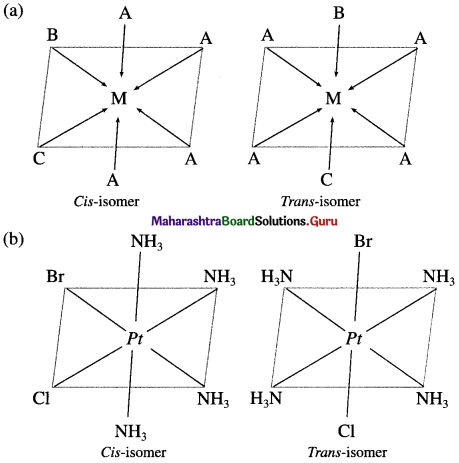Question 27.
Define : (1) Optical isomerism (2) Optical isomers.
(1) Optical isomerism : The phenomenon of isomerism in which different coordination compounds having same molecular formula have different optical activity is called optical isomerism.

(2) Optical isomers : Different coordination compounds having same molecular formula but different optical activity
are called optical isomers.

Question 28.
Explain : (1) Plane polarised light (2) Optical activity.
(1) Plane polarised light : A monochromatic light having vibrations only in one plane is called a plane polarised light. This light is obtained by passing monochromatic light through NICOL prism.

(2) Optical activity : A phenomenon of rotating a plane of a plane polarised light by an optically active substance is
called optical activity. This substance is said to be optically active.Question 29.
Explain : (1) Dextrorotatory substance (2) Laevorotatory substance. (1 mark each)

1. Dextrorotatory substance : An optically active substance which rotates the plane of a plane polarised light to right hand side is called dextrorotatory or d isomer denoted by d.
2. Laevorotatory substance : An optically, active substance which rotates the plane of a plane polarised light to the left hand side is called laevorotatory or l isomer and denoted by l.

Question 30.
What are the conditions for the optical isomerism in coordination compounds?

• Optical isomerism is exhibited by those coordination compounds which possess chirality.
• There should not be the presence of element of symmetry which makes the complex optically inactive.
• The mirror images of the complex molecule or ion must be non-superimposable with the molecule or ion. B

Question 31.
What are enantiomers?
Enantiomers : The two forms of the optical active complex molecule which are mirror images of each other are called enantiomers.

There are two forms of enantiomers, d form and l form.

Question 32.
Draw diagrams for the optical isomers of a complex, [Co(en)3]3+.
The complex [Co(en)3]3+ has two optical isomers.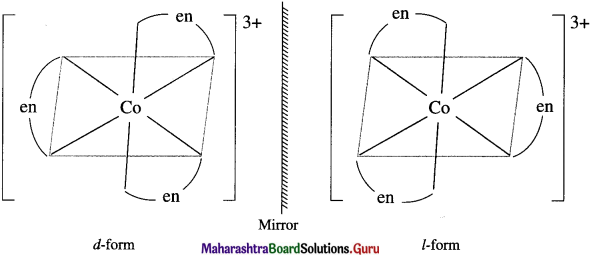Question 33.
Explain the optical isomerism in the octahedral complex with two symmetrical bidentate chelating ligands.
The octahedral complexes of the type [M(AA)2Q2]”±, in which two symmetrical bidentate chelating ligands like AA and two monodentate ligands like a are coordinated to the central metal atom or ion exhibit optical isomerism and two optical isomers d and l can be resolved. For example, [Pt Cl2(en)2]2.

The cis-form is unsymmetrical and optically active while the trans-form is symmetrical and hence optically inactive. The optical isomers of cis-form (d and ) of this complex along with trans-form are shown below,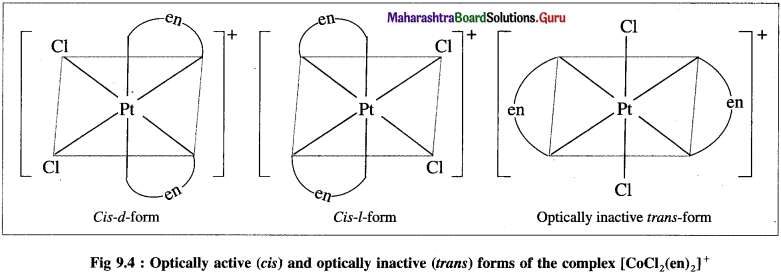Question 34.
When are optical isomers called chiral?
When the mirror images of optical isomers of the complex are nonsuperimposable they are said to be chiral. For example, [Co(en)2(NH3)2]3+.

Question 35.
Define and explain ionisation isomerism.
Ionisation isomerism : The phenomenon of isomerism in the metal complexes in which there is an exchange of ions between coordination (or inner) sphere and outer sphere is known as ionisation isomerism.

Explanation :

• Ionisation isomers have same molecular formula but different arrangement of ions in the inner sphere and outer sphere in the complex,
• Hence on ionisation, these ionisation isomers produce different ions in the solution. This ionisation isomerism is also called ion-ion exchange isomerism.

Examples:
(A) [CO(NH3)4CI2]Br and (B) [Co(NH3)4CIBr] Cl

Ionisation :
(A) [CO(NH3)4CI2] Br ⇌ [CO(NH3)4CI2]+ + Br-
(B) [Co(NH3)4CIBr]Cl ⇌ [CO(NH3)4ClBr]+ + CP
In the isomers (A) and (B), there is an exchange of ions namely Br and CI.Question 36.
OR
What is linkage isomerism ? Explain with an example.
(1) Linkage isomerism : The phenomenon of isomerism in which the coordination compounds have same metal atom or ion and same ligand but bonded through different donor atoms or linkages is known as linkage isomerism.

(2) Linkage isomers : The coordination compounds having same metal atom or ion and ligand but bonded through different donor atoms or linkages are called linkage isomers.
For example : Nitro complex [CO(NH3)5NO2]CI2 = (Yellow) and nitrito complex [CO(NH3)5ONO]CI2 (Red)

Question 37.
Explain linkage isomers with NO2 group as a ligand.
(1) Nitro group (NO2) is an ambidentate ligand. NO2 group may link to central metal atom, through N or O.
(2) The two linkage isomers are, [CI: → Ag ← : NO2] and [CI: → Ag ← O-NO]
Choloronitroargentate(I) ion and Chloronitritoargentate(I) ion

Question 38.
Write linkage isomers of a complex having constituents Co3+, 5NH3 and NO2.
(i) NO2 is an ambidentate ligand which can be linked through N or O.
(ii) The linkage isomers are as follows :
(a) [CO(NH3)5(NO2)]2+ Pentaamminenitrocobalt(III) ion
(b) [CO(NH3)5(ONO)]2+ Pentaamminenitritocobalt(III) ion

Question 39.
Define: (1) Coordination isomerism (2) Coordination isomers.
(1) Coordination isomerism : The phenomenon of isomerism in the ionic coordination compounds having the same molecular formula but different complex ions involving the interchange of ligands between cationic and anionic spheres of different metal. ions is called coordination isomerism.

(2) Coordination isomers : The ionic coordination compounds having same molecular formula but different complexions duc to interchange of ligands between cationic and anionic spheres of different metal ions are called coordination isomers.

For example,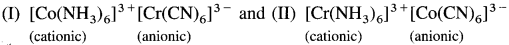Question 40.
Give three examples of coordination isomers. (I mark each)

• [Cu(NH3)4] [PICI4] and I Pt(NH3)4] [ICuCl44]
• [Cr(NH3)6] [Cr(CN)6] and [Cr(NH3)4(CN)2] [Cr(NH3)2(CN)4]
• [Cr(NH3)6] [Cr(SCN)6] and [Cr(NH3)4(SCN)2] [Cr(SCN)4(NH3)2]

Question 41.
Define Solvate or Hydrate isomerism.
Solvate ate or Hydrate isomerism : The phenomenon of isomerism in the coordination compounds arising due to the exchange of solvent or H2O molecules inside the coordination sphere and outer sphere of the complex is known as solvate or hydrate isomerism.

Question 42.
Define solvate or hydrate isomers.
OR
What are hydrate isomers? Explain with examples.
Solvate or Hydrate isomers : The coordination compounds having the same molecular formula but differ in the number of solvent or H2O molecules inside the coordination sphere and outer sphere of the complexes are called solvate or hydrate isomers.

For example : [Cr(H2O)6] CI3; [Cr(H2O)5CI]CI2 H2O; and [Cr(H2O)4CI2] CI 2H2O.

Question 43.
A coordination compound has the formula COCI3 6H2O. Write the hydrate isomers of the complex.
The possible hydrate isomers of the coordination compounds having molecular formula COCI3 6H2O are as follows :
(1) [CO(H2O)6]CI3;
(2) [CO(H2O)5CI]CI2 H2O
(3) [CO(H2O)4CI2] Cl – 2H2O
(4) [Co(H2O)3 CI3] 3H2O.Question 44.
Explain the steps involved in describing the bonding in coordination compounds using valence bond theory.

• Vacant d-orbitais of metal ion form coordination bonds with ligands.
• s, p orbitais along with vacant d-orbitais of metal ion take part in hybridisation.
• The number of vacant hybrid orbitais formed is equal to number of hybridising orbitais which is equal to the number of ligand donor atoms or coordination number of the metal.
• The metal-ligand coordination bonds are formed by the overlap between the vacant hybrid orbitais of metal and the filled orbitais of the ligands.
• The hybrid orbitais used by the metal ion point in the direction of the ligands.
• When inner (n – 1)d orbitais of metal ion are used in the hybridisation then the complex is called (a) inner orbital complex while when outer nd orbitais are used, complexes are called (b) outer orbital complexes. .

Question 45.
Explain the steps involved ¡n the metal-ligand bonding.

• Find the oxidation state of central metal ion in the complex.
• Write the valence shell electronic configuration of metal ion.
• From the formula of the complex determine the number of ligands and find the number of metal ion orbitais required for bonding.
• Find the orbitais of metal ion available for hybridisation and the type of hybridisation involved.
• Represent the electronic configuration of metal ion after hybridisation.
• Exhibit filling of hybrid orbitais after complex formation.
• Determine the nunther of unpaired electrons and predict magnetic property of the complex.
• Find whether the complex is low spin or high spin (applicable for octahedral complexes with d4 or d8 electronic configuration.)

Question 46.
What are the salient features of valence bond theory (VBT)?
The salient features of valence bond theory (VBT) are as follows :

1. According to this theory, a central metal atom or ion present in a complex provides a definite number of vacant orbitals (s, p, d and) to accommodate the electrons from the ligands for the formation coordinate bonds with the metal ion atom.
2. The number of vacant orbitals provided by the central metal atom or ion is the same as the coordination number of the metal. For example : Cu2+ provides 4 vacant orbitals in the complex. [Cu(NH3)4]2+.
3. The vacant orbitals of metal atom or ion undergo hybridisation forming the same number of hybridised orbitals, since the bonding with the hybrid orbitals is stronger.
4. Each ligand has one or more orbitals containing one or more lone pairs of electrons.
5. The shape or geometry of the complex depends upon the type of hybridisation of the metal atom.
6. When inner orbitals namely (n – 1) d orbitals in transition metal atom or ion hybridise, the complex is called inner complex and when outer orbitals i.e., nd orbitals hybridise then the complex is called outer complex.
7. When the central metal atom or ion in the complex contains one or more unpaired electrons the complex is
paramagnetic while if all the electrons are paired, the complex is diamagnetic.

Question 47.
What is the spin pairing process in the coordination compound?
When the ligands approach the metal atom or ion for the formation of a complex, they influence the valence electrons of metal atom or ion. Accordingly the ligands are classified as (A) strong ligands and (B) weak ligands.

(A) Strong ligands :

• They cause the pairing of unpaired electrons present in the metal atom or ion.
• Spin pairing process :
• The process of pairing of unpaired electrons in metal atom or ion due to the presence of ligands in the complex is called spin pairing process.
• This spin pairing process decreases the number of unpaired electrons and hence decreases the paramagnetic character of the complex.
• The strong ligands also promote the outer ns electrons to the vacant inner (n – 1)d orbitals.

(B) Weak ligands : The weak ligands have no effect on the electrons in the valence shell of a metal atom or ion.
Strong ligands : CO, CN, ethylenediammine (en), NH3, EDTA, etc.
Weak ligands : CI, I, OH, etc.

[Note : If a complex has n number of unpaired electrons then the magnetic moment, μ is given by ‘spin only’ formula μ = n(n + 2) B.M. where B.M. (Bohr Magneton) is the unit of magnetic moment. Hence from the magnitude of p, the number of unpaired electrons in the complex and its structure can be evaluated.]Question 48.
Explain the structure of octahedral complex, [CO(NH3)6]3+ on the basis of valence bond theory.
(1) Hexaamminecobalt(III) ion, [CO(NH3)6]3+ is a cationic complex, the oxidation state of cobalt is + 3 and the coordination number is 6.

(2) Electronic configuration : 27CO [Ar]18 3d7 4s2
Electronic configuration : Co3+ [Ar]18 3d6 4s° 4p°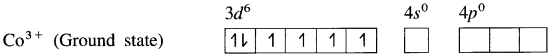(3) Since NH3 is a strong ligand, due to spin pairing effect, all the four unpaired electrons in 3d orbital are paired giving two vacant 3d orbitals.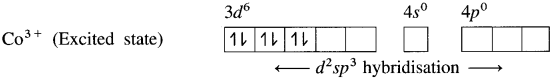(4) Since the coordination number is Co3+ ion gets six vacant orbitais by hybridisation of two 3d vacant orbitais, One 4s and three 4p orbitais forming six d2sp3 hybrid orbitais giving octahedral geometly. It is an inner complex.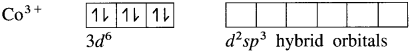(5) 6 lone pairs of electrons from 6NH3 ligands are accommodated in the six vacant d2sp3 hybrid orbitals. Thus six hybrid orbitals of Co3+ overlap with filled orbitals of NH3 forming 6 coordinate bonds giving octahedral geometry to the complex.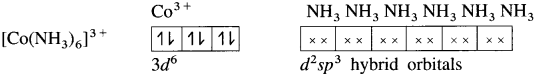Since the complex has all electrons paired, it is diamagnetic.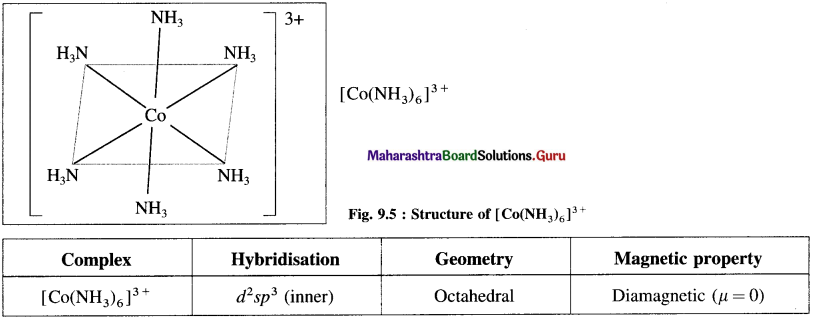Question 49.
Explain the geometry of [CoF6]3- on the basis of valence bond theory.
(1) Hexafluorocobaltate(III) ion, [CoF]3- is an anionic complex, the oxidation state of cobalt is +3 and the coordination number is 6.
(2) Electronic configuration : 27Co [Ar]18 3d7 4s2 4p° 4d°
Electronic configuration : Co3+ [Ar]18 3d6 4s° 4p° 4d°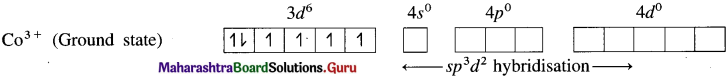(3) Since F is a weak ligand, there is no spin pairing effect and Co3+ possesses 4 unpaired electrons.
(4) Since the coordination number is 6, the Co3+ ion gets six vacant orbitals by hybridisation of one 45 orbital, three 4p orbitals and two 4d orbitals forming six sp3d2 hybrid orbitals giving octahedral geometry.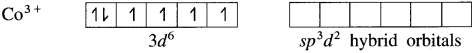(5) 6 lone pairs of electrons from 6F ligands are accommodated in the six vacant sp3d2 hybrid orbitals. Thus six hybrid orbitals of Co3+ overlap with filled orbitals of F forming 6 coordinate bonds giving octahedral geometry to the complex. It is an outer complex.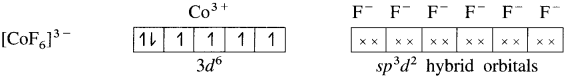As the complex has 4 unpaired electrons it is paramagnetic.
Magnetic movement μ is,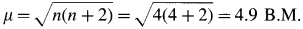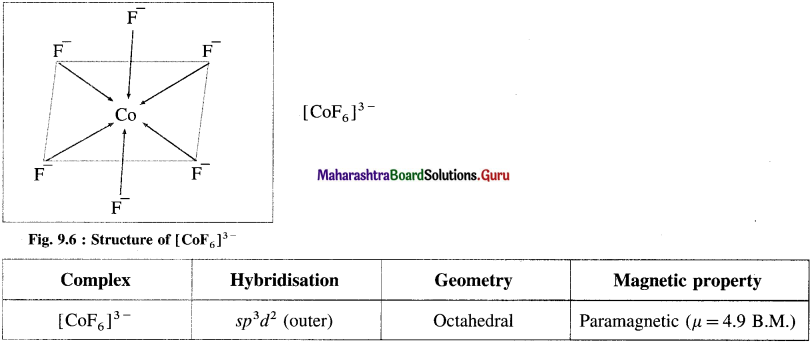Question 50.
Explain the structure of tetrachloronickelate(II) [NiCI]2- on the basis of valence bond theory.
(1) Tetrachloronickelate(II) ion is an anionic complex, oxidation state of Ni is +2 and the coordination number is 4.
(2) Electronic configuration : 28Ni [Ar]18 3d8 4s2 4p°
Electronic configuration : Ni2+ [Ar]18 3d8 4s° 4p°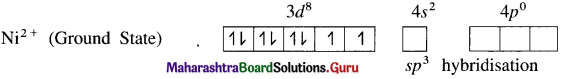(3) Since the coordination number is 4, it gets 4 vacant hybrid orbitals by sp3 -hybridisation of one 4s and three 4p orbitals giving tetrahedral geometry to the complex.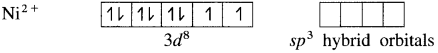(4) As Cl is a weak ligand, 2 unpaired electrons in Ni2+ remain undisturbed.
(5) 4 lone pairs of electrons from 40 ligands are accommodated in the vacant four sp3 hybrid orbitals. Thus four sp3 hybrid orbitals of Ni2+ overlap with filled orbitals of Clforming 4 coordination bonds, giving tetrahedral geometry to the complex.
Since the complex has 2 unpaired electrons, it is paramagnetic.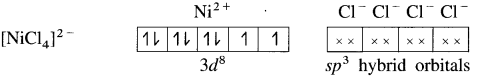Magnetic moment $$\mu \text { is, } \mu=\sqrt{n(n+2)}=\sqrt{2(2+2)}=2.83 \text { B.M. }$$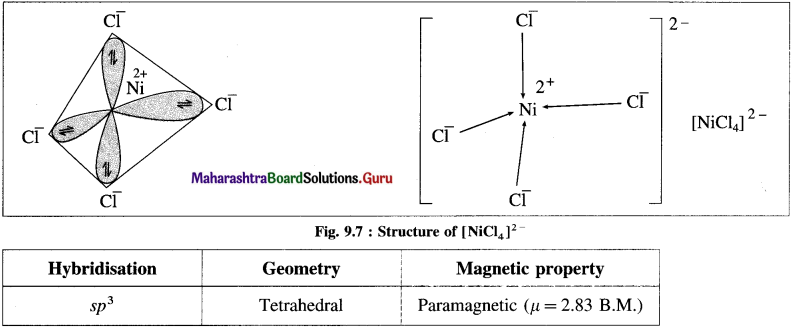Question 51.
Explain the structure of [Ni(CN)4]2- on the basis of valence bond theory.
(1) Tetracyanonickelate (II) ion, INi(CN)2]2- is an anionic complex, oxidation state of Ni is + 2 and the coordination number is 4.
(2) Electronic configuration : 22Ni [Ar]18 3d8 4s2 4p°
Electronic configuration: Ni2+ [Ai]18 3d8 4s° 4p°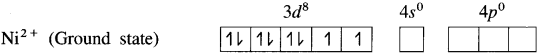(3) Since CN is a sa-ong ligand, one of the unpaired electrons in 3d orbital is promoted giving two paired electrons and one vacant 3d orbital.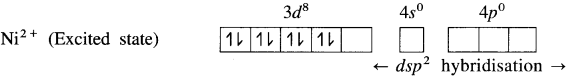(4) Since the coordination number is 4, Ni2+ gets 4 vacant hybrid orbitais by hybridisation of one 3d, one 4s and two 4p orbitais forming four dsp2 hybrid orbitaIs. This has square planar geometry.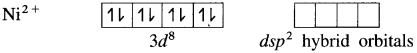(5) 4 lone pairs from 4CN ligands are accommodated in the vacant four dsp2 hybrid orbitais. Thus four dps2 hybrid orbitais of Ni2+ overlap with filled orbitais of CN forming 4 coordinate bonds giving square planar geometry to the complex. It is an inner complex.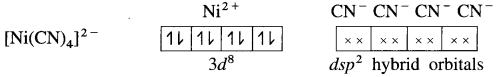Since the complex ion has all electrons paired, it is diamagnetic.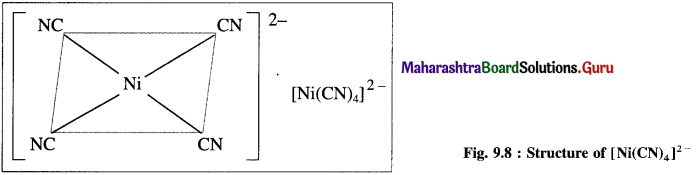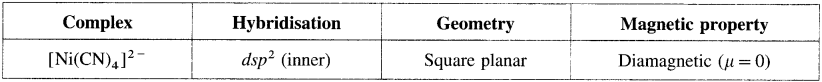Question 52.
What are the limitations of valence bond theory?
In case of the coordination compounds, the valence bond theory has the following limitations :
(1) It cannot explain the spectral properties (colours) of the complex compounds.
(2) Even if the magnetic moments can be calculated from the number of unpaired electrons, it cannot explain the magnetic moment arising due to orbital motion of electrons.
(3) It cannot explain why the metal ions with the same oxidation state give inner complexes and outer complexes with different ligands.
(4) In every complex, it cannot explain magnetic properties based on geometry of the complex.
(5) Quantitative interpretations of thermodynamic and kinetic stabilities of the coordination compounds cannot be accounted.
(6) The complexes with weak field ligands and strong field ligands cannot be distinguished.
(7) It cannot predict the tetrahedral and square planar geometry of complexes with coordination number 4.
(8) The order of reactivity of inner complexes of d3, d4, d5 and d6 metal ions cannot be explained.
(9) It cannot explain the rates and mechanisms of reactions of the coordination compounds.

Question 53.
What are the assumptions of Crystal Field Theory (CFT)?
Bethe and van Vleck developed Crystal Field Theory (CFT) to explain various properties of coordination compounds. The salient features of CFT are as follows :

1. In a complex, the central metal atom or ion is surrounded by various ligands which are either negatively charged ions (F, CI, CN, etc.) or neutral molecules (H2O, NH3, en, etc.) and the most electronegative atom in them points towards central metal ion.
2. The ligands are treated as point charges involving purely electrostatic attraction between them and metal ion.
• The central metal ion has five, (n – 1)d degenerate orbitals namely dxy, dyz, dzx, d(x2 – y2) and dz2.
• When the ligands approach the metal ion, due to repulsive forces, the degeneracy of <i-orbitals is destroyed and they split into two groups of different energy, t2g and eg orbitals. This effect is called crystal field splitting which depends upon the geometry of the complex.
• The T-orbitals lying in the direction of ligands are affected to a greater extent while those lying in between the ligands are affected to a less extent.
• Due to repulsion, the orbitals along the axes of ligands acquire higher energy while those lying in between the ligands acquire less energy.
• Hence repulsion by ligands give two sets of split up orbitals of metal ion with different energies.
• The energy difference between two sets of d-orbitais after splitting by ligands is called crystal field splitting energy (CFSE) and represented by Δ0 or by arbitrary term 10Dq. The value of Δ or 10Dq depends upon the geometry of the complex.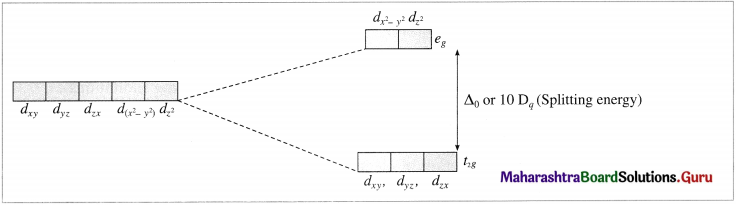3. The electrons of metal ion occupy the split d-orbitais according to Hund’s rule. aufbau principle and those orbitais with minimum repulsion and the farthest away from the ligands.
4. CFI’ does not account for overlapping of orbitais of central metal ion and ligands, hence does not consider covalent nature of the complex.
5. From the crystal field stability energy, the stability of the complexes can he known.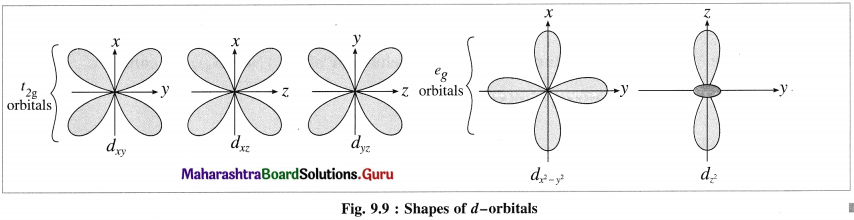Question 54.
What is crystal field splitting?
The splitting of five degenerate d-orbitals of the transition metal ion into different sets of orbitals (to2g and eg) having different energies in the presence of ligands in the complex is called crystal field splitting.

Question 55.
What is crystal field stabilisation energy?
Crystal field stabilisation energy (CFSE) It is the change in energy achieved by preferential filling up of the orbitals by electrons in the complex of metal atom or ion.

CFSE is expressed as a negative .quantity i.e., CFSE < 0. Higher the negative value more is the stability of the complex. m

Question 56.
Explain the factors affecting Crystal Field Splitting parameter (Δ0).
Crystal Field Splitting parameter (Δ0) depends on. (a) Strength of the ligands and (b) Oxidation state of the metal.

(a) Strength of the ligands : Since strong field ligands like CN, en, etc. approach closer to the central metal ion, it results in a large crystal field splitting and hence Δ0 has higher values.
(b) Oxidation state of the metal A metal ion with the higher positive charge draws the ligands closer to it which results in large separation of t2g and eg set of orbitals. The complexes involving metal ions with low oxidation state have low values of Δ0. For example [Fe(NH3)6]3+ has higher Δ0 than [Fe(NH3)6]2+. HQuestion 57.
Explain the octahedral geometry of complexes using crystal field theory.
(1) In an octahedral complex [MX6]n+, the metal atom or ion is placed at the centre of regular octahedron while six ligands occupy the positions at six vertices of the octahedron.

(2) Among five degenerate d-orbitals. two orbitals namely dx2 – y2 and dz2 are axial and have maximum electron density along the axes, while remaining three c-orbitals namely dxy, dyz and dyz are planar and have maximum electron density in the planes and in-between the axes.

(3) Hence, when the ligands approach a metal ion, the orbitals dx2 – y2 and dz2 experience greater repulsion and the orbitals dzy, dyz and dzx experience less repulsion.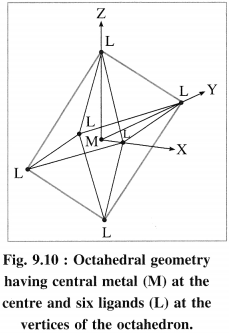(4) Therefore the energy of dx2 – y2 and dz2 increases while the energy of dxy, dyz and dzx decreases and five d-orbital lose degeneracy and split into two point groups. The orbitals dxy, dyz and dyz form t2g group of lower energy while dx2 – y2 and dz2 form e group of higher energy.

Thus t2g has three degenerate orbitals while eg has two degenerate orbitals.

(5) Experimental calculations show that the energy of t2g orbitals is lowered by 0.4Δ0 or 4Dq and energy of eg. orbital is increased by 0.6 Δ0 or 6Dq Thus energy difference between t2g and eg orbitals is Δ0 or 10Dg which is crystal field splitting energy.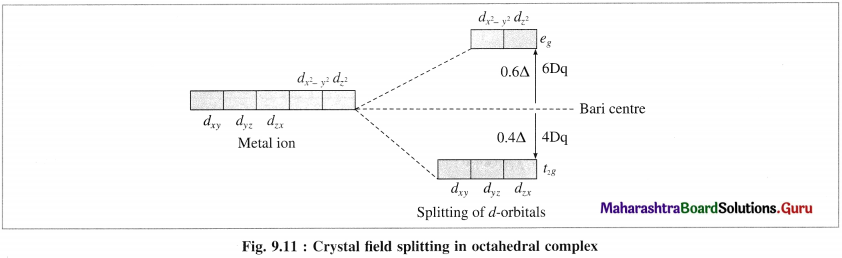(6) CFSF increases with the increasing strength of ligands and oxidation stale of central metal ion.

Question 58.
Explain the tetrahedral geometry of complexes using crystal field theory.
(1) In the tetrahedral complex, [MX4], the metal atom or ion is placed at the centre of the regular tetrahedron and the four ligands, are placed at four corners of the tetrahedron.

(2) The ligands approach the central metal atom or ion in-between the three coordinates x, y and The orbitals dxy, dyz and d are pointed towards ligands and experience greater repulsion while the axial orbitals dx2y2 and dz2 lie in-between metal-ligand bond axes and experience comparatively less experience.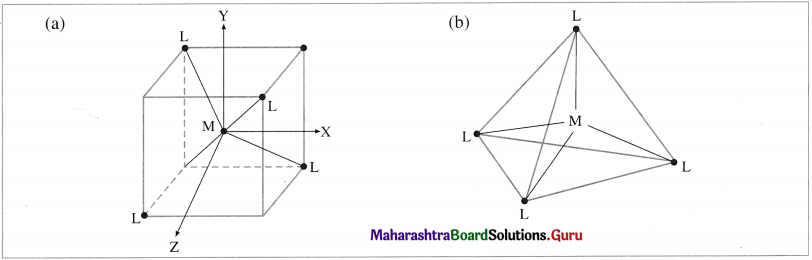Fig. 9.12 (a) and (b) : Tetrahedral geometry hasing central metal atoll) (M) at the centre and four ligands (L) al the four corners(3) Therefore energy of dxy, dyz and ddzx orbitals is increased while that of dx2y2 and dz2 is lowered. Hence 5d-orbitals lose their degeneracy and split into two point groups, namely f2? of higher energy (dxy, dyz and dzx) and eg of lower energy (dx2 – y2 and dz2).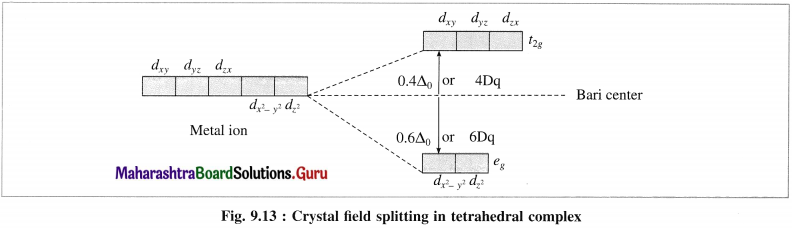(4) The experimental calculations show that the energy of t2g orbitals is increased by 0.4 Δ0 or 4Dq and energy of eg is lowered by 0.6 Δ0 or 6Dg. Thus energy difference between t2g and eg orbitals is Δ0 or 10Dg which is crystal field splitting energy (CFSE).

(5) This explains that the entry of each electron in eg orbitals, stabilises the complex by 0.6 Δ0 or 6Dq While the entry of each electron in t2g orbitals destabilises the tetrahedral complex by 0.4 Δ0 or 4Dg.

(6) In case of strong field ligands, the electrons prefer to pair up in eg orbitals giving low spin (LS) complexes while in case of weak held ligands, the electrons prefer to enter higher energy t2g orbitals giving more unpaired electrons and hence form high spin (HS) complexes.

Table 9.4 : Properties of complexes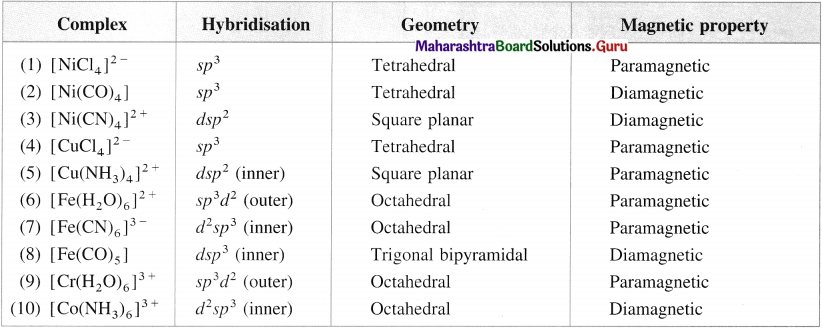Question 59.
Give valence bond description for the bonding in the complex [VCI4]. Draw box diagrams for free metal ion. Which hybrid prbitals are used by the metal? State the number of unpaired electrons.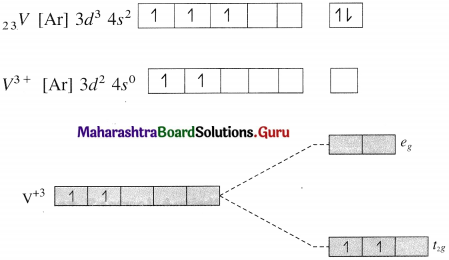Since CI is a weak ligand, there is no pairing of electrons.
Number of unpaired electrons = 2
Type of hybridisation = sp3

Geometry of complex ion = Tetrahedral
The complex ion is paramagnetic.Question 60.
Write a note on colour in coordination compounds.

• A large number of coordination compounds show wide range of colours due to d – d transition of electron and this can be explained by crystal field theory (CFT).
• The complex absorbs the light in one visible region (400 nm to 700 nm) and transmits the light in different visible region giving complementary colour.
• Consider an octahedral purple coloured complex of [Ti(H2O)6]3+ which absorbs green light and transmits purple colour. Similarly [Cu(H2O)6]2+ absorbs the light in the red region of radiation spectrum and transmits in the blue region, hence the complex appears blue.
• The absorption of light arises due to d-d transition of electron from lower energy level (t2g) to higher energy level (eg) in octahedral complex.
• The energy required for transition depends upon crystal field splitting energy Δ0. If Δ0 = ΔE, then the energy of an absorbed photon (hv) is $$\Delta E=h v=\frac{h c}{\lambda}$$ where λ, v and c are wavelength, frequency and velocity of the absorbed light.
• Higher the magnitude of Δ0 or ΔE, higher is the frequency or lower is the wavelength of the absorbed radiation.
• Since Δ0 depends upon nature of metal atom or ion, its oxidation state, nature of ligands and the geometry of the complex, different coordination compounds have different colours.

Question 61.
Explain the purple colour of the complex, [Ti(H2O)6]3+ with the help of crystal field theory.
(1) [Ti(H2O)6]3+ is an octahedral complex, oxidation state of titanium is +3 (Ti3+) and the coordination number is 6.

(2) Electronic configuration = 22Ti [Ar]18 3d2 4s2
Electronic configuration = Ti3+ + [Ar]18 3d1
OR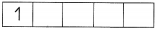(3) According to crystal field theory, 3d orbitals undergo crystal field splitting giving higher energy eg, two orbitals and lower energy t2g, three orbitals.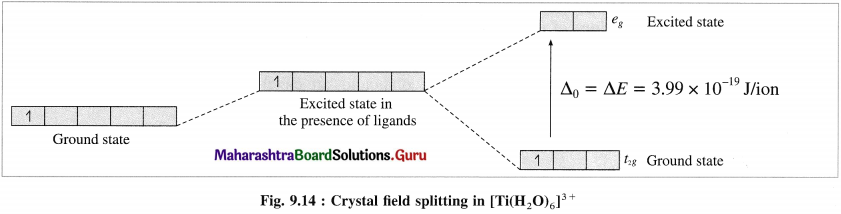(4) The crystal field splitting energy (CFSE), Δ0 is found to be 3.99 x 10-19 J/ion from the spectrochemical studies.

(5) The absorption of radiation of wavelength λ or frequency v results in the transition of one unpaired electron from Photon energy t2g orbital to eg orbital.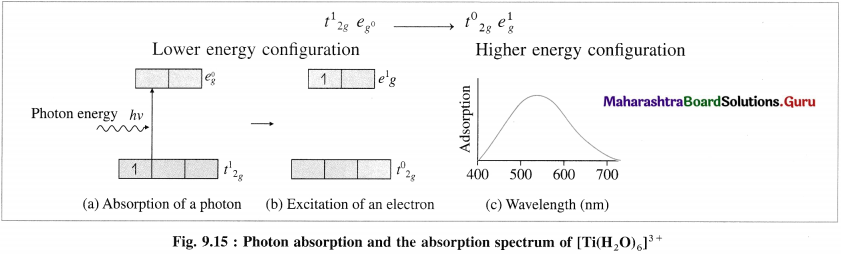(6) The wavelength of the absorbed radiation will be.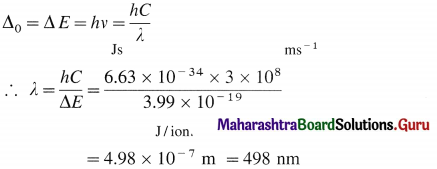(7) Hence the complex [Ti(H2O)6]3+ absorbs the green radiation of wavelength 498 nm in the visible region and transmits the complementary purple light. Therefore the complex is purple coloured.

Question 62.
An octahedral complex absorbs the radiation of wavelength 620 nm. Find the crystal field splitting energy.
Crystal field splitting energy Δ0 is given by,Question 63.
What are the applications of coordination compounds ?
(1) In biology : Several biologically important natural compounds are metal complexes which play an important role in number of processes occurring in plants and animals.

For example, chlorophyll in plants is a complex of Mg2+ ions, haemoglobin in blood is a complex of iron, vitamin B12 is a complex of cobalt.

(2) In medicine : The complexes are used on a large scale in medicine. Many medicines in the complex form are more stable, more effective and can be assimilated easily.

For example, platinum complex [Pt(NH3)2CI2] known as cisplatin is effectively used in cancer treatment. EDTA is used to treat poisoning by heavy metals like lead.

(3) To estimate hardness of water :

• The hardness of water is due to the presence Mg2+ and Ca2+ ion in water.
• The strong field ligand EDTA forms stable complexes with Mg2+ and Ca2+. Hence these ions can be removed by adding EDTA to hard water.

Similarly these ions can be selectively estimated due to the difference in their stability constants.

(4) Electroplating : This involves deposition of a metal on the other metal. For smooth plating, it is necessary to supply continuously the metal ions in small amounts.

For this purpose, a solution of a coordination compound is used which dissociates to a very less extent. For example, for uniform and thin plating of silver and gold, the complexes K[Ag(CN)2] and K[Au(CN)2] are used.

Multiple Choice Questions

Select and write the most appropriate answer from the given alternatives for each subquestion :

Question 1.
The coordination number of cobalt in the complex [Co(en)2Br2]CI2 is
(a) 4
(b) 5
(c) 6
(d) 7
(c) 6

Question 2.
EDTA combines with cations to form
(a) chelates
(b) polymers
(c) clathrates
(d) non-stoichiometric compounds
(a) chelates

Question 3.
Which one of the following compounds can exhibit coordination isomerism?
(a) [Co(en)2CI2]Br
(b) [CO(NH3)6] [Cr(CN)6]
(c) [Co(en)3]CI3
(d) [CO(NH3)5NO2]CI2
(b) [CO(NH3)6] [Cr(CN)6]

Question 4.
Which of the following compounds can exhibit linkage isomerism?
(a) [Co(en)3]CI3
(b) [Co(en)2CI2]CI
(c) [Co(en)2NO2Br]CI
(d) [Co(NH3)5CI]Br2
(c) [Co(en)2NO2Br]CI

Question 5.
Oxidation number of cobalt in K[COCI4] is
(a) +1
(b) -1
(c) +3
(d) -3
(b) -1Question 6.
The correct structure of [Cr(H2O)6]3+ is ……………………
(a) octahedral
(b) tetrahedral
(c) square pyramidal
(d) trigonal bipyramidal
(b) tetrahedral

Question 7.
Amongst the following ions which one has the highest paramagnetism?
(a) [Cr(H2O)6]3+
(b) [Fe(H2O)6]2+
(c) [CU(H2O)6]2+
(d) [Zn(H2O)6]2+
(b) [Fe(H2O)6]2+

Question 8.
The geometry of [Ni(CN)4]3- and [NiCI4]-2 are
(a) both tetrahedral
(b) both square planar
(c) tetrahedral and square planar respectively
(d) square planar and tetrahedral respectively
(d) square planar and tetrahedral respectively

Question 9.
The complex cis-[Pt(NH3)2CI2] is used in treatment of cancer under the name.
(a) Aspirin
(b) Eqanil
(c) cisplatin
(d) transplatin
(c) cisplatin

Question 10.
[CO(NH3)6]3+ is an orbital complex and is in nature.
(a) inner, paramagnetic
(b) inner, dimagnetic
(c) outer, paramagnetic
(d) outer, dimagnetic
(b) inner, dimagnetic

Question 11.
The IUPAC name of [Ni(Co)4] is
(a) tetra carbonyl nickel (O)
(b) tetra carbonyl nickel (II)
(c) tetra carbonyl nickelate (O)
(d) tetra carbonyl nickelate (II)
(a) tetra carbonyl nickel (O)

Question 12.
The number of ions produced by the complex [CO(NH3)4CI2] Cl is
(a) 1
(b) 2
(c) 3
(d) 4
(b) 2

Question 13.
The dimagnetic species is
(a) [Ni(CN)4]2-
(b) [NiCl4]2-
(c) [CoCI4]2-
(d) [CoF6]2-
(a) [Ni(CN)4]2-

Question 14.
Which one of the following is an inner orbital complex as well as diamagnetic in behaviour (Atomic no. Zn = 30, Cr = 24, Co = 27, Ni = 28)
(a) [Zn(NH3)6]2+
(b) [Cr(NH3)6]3+
(c) [CO(NH3)6]3+
(d) [Ni(NH3)6]2+
(c) [CO(NH3)6]3+Question 15.
Among [Ni(Co)J, [Ni(CN)4]2A [NiClJ2- Species, the hybridisation states at the Nickel atom are respectively
(a) sp3, dsp2, sp3
(b) sp3, dsp2, dsp2
(c) dsp2, sp3, sp3
(d) sp3, sp3, dsp2
(a) sp3, dsp2, sp3

Question 16.
The strongest ligand in the following is
(a) CN
(b) Br
(c) HO
(d) F
(a) CN

Question 17.
Magnetic moment of (NH4)2 (MnBr4) is BM
(a) 5.91
(b) 4.91
(c) 3.91
(d) 2.91
(a) 5.91

Question 18.
The complex which violates EAN rule is
(a) Fe(CO)5
(b) [Fe(CN)6]3-
(c) Ni(CO)4
(d) [Zn(NH3)4]CI2
(b) [Fe(CN)6]3-

Question 19.
EDTA is a ligand of the type
(a) bidentate
(b) tridentate

Question 20.
The cationic complex among the following is
(a) K3[Fe(CN)6]
(b) Ni(CO)4
(c) K2HgI4
(d) [CO(NH3)6]CI2
(d) [CO(NH3)6]CI2

Question 21.
If Z is the atomic number of a metal, X is number of electrons lost forming metal ion and Y is the number of electrons from the ligands then EAN is
(a) Z + X + Y
(b) X – Z + Y
(c) Z – X + Y
(d) X + Z – Y
(c) Z – X + Y

Question 22.
Octahedral complex has hybridisation,
(a) dsp2
(b) d3sp3
(c) dsp3
(d) d2sp3
(d) d2sp3Question 23.
Inner complex has hybridisation,
(a) d2sp3
(b) sp3d2
(c) sp3d
(d) sp3d3
(a) d2sp3

Question 24.
The number of unpaired electrons in [CO(NH3)6]3+ is
(a) 0
(b) 1
(c) 2
(d) 4
(a) 0

Question 25.
The number of unpaired electrons in [NiClJ2- and [Ni(CN)4]2_ are respectively,
(a) 2, 2
(b) 2, 0
(c) 0, 0
(d) 1, 2
(b) 2, 0

Question 26.
Among the following complexes, the highest magnitude of crystal field stabilisation energy will be for [Co(H2O)6]3+, [CO(CN)6]3-, [Co(NH3)6]3+, [CoF6]3-
(a) [Co(H2O)6]3+
(b) [CO(CN)6]3-
(c) [Co(NH3)6]3+
(d) [CoF6]3-
(b) [CO(CN)6]3-

Question 27.
The number of unpaired electrons in a low spin octahedral complex ion of d1 is
(a) 0
(b) 1
(c) 2
(d) 3
(b) 1

Question 28.
The number of unpaired electrons in a high spin octahedral complex ion of d7 is
(a) 0
(b) 1
(c) 2
(d) 3
(d) 3

Question 29.
Ligand used in the estimation of hardness of water is
(a) EDTA
(b) DBG
(c) chloride
(d) bromo
(a) EDTA

Question 30.
Which of the following complexes will give a white precipitate on treatment with a solution of barium nitrate?
(a) [Cr(NH3)4SO4] CI
(b) [CO(NH3)4CI2] NO2
(c) [Cr(NH3)4CI2] SO4
(d) [CrCI3(H2O)4]CI
(c) [Cr(NH3)4CI2] SO4Question 31.
What is effective atomic number of Fe (z = 26) in [Fe(CN)6]4-?
(a) 12
(b) 30
(c) 26
(d) 36